# Special Functions in Wolfram|Alpha

June 15, 2010 —Comments Off

Wolfram|Alpha computes things. While the use of computations to predict the outcomes of scientific experiments, natural processes, and mathematical operations is by no means new (it has become a ubiquitous tool over the last few hundred years), the ease of use and accessibility of a large, powerful, and ever-expanding collection of such computations provided by Wolfram|Alpha is.

Virtually all known processes occur in such a way that certain functionals that describe them become extremal. Typically this happens with the action for time dependent processes and quantities such as the free energy for static configurations. The equations describing the extremality condition of a functional are frequently low-order ordinary and/or partial differential equations and their solutions. For example, for a pendulum: Frechet derivative of Integrate[x'[t]^2/2 – Cos[x[t]], {t, -inf, inf}] wrt x[tau]. Unfortunately, if one uses a sufficiently realistic physical model that incorporates all potentially relevant variables (including things like friction, temperature dependence, deformation, and so forth), the resulting equations typically become complicated—so much so that in most cases, no exact closed-form solution can be found, meaning the equations must be solved using numerical techniques. A simple example is provided by free fall from large heights: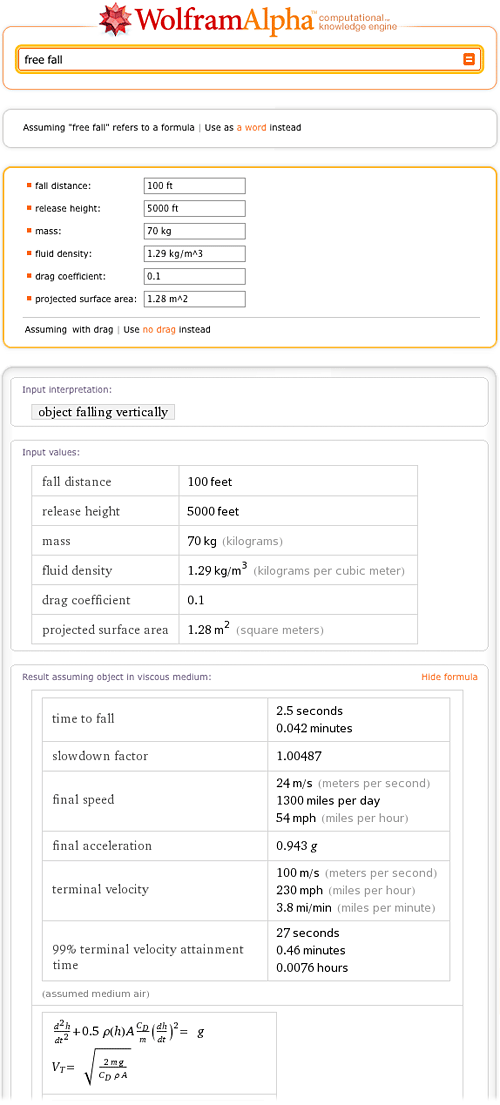On the other hand, some systems, such as the force of a simple spring, can be described by formulas involving simple low-order polynomial or rational relations between the relevant problem variables (in this case, Hooke’s law, F = k x):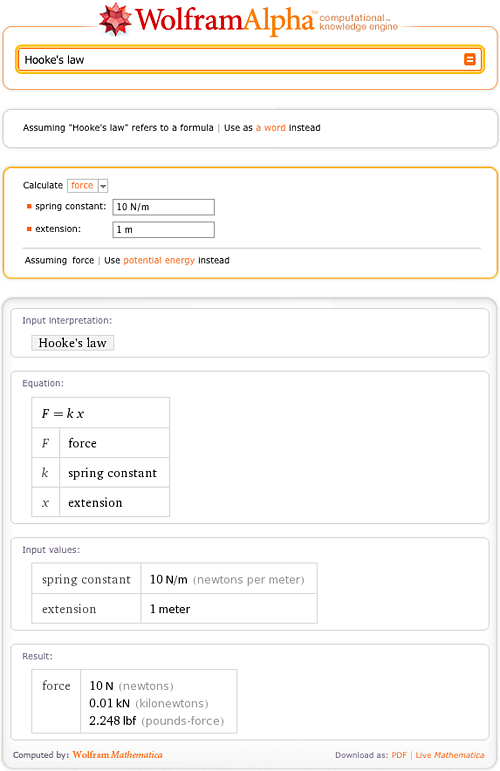Over the last 200+ years, mathematicians and physicists have found a large, fascinating, and insightful world of phenomena that can be described exactly using these so-called special functions (also commonly known as “the special functions of mathematical physics”), the class of functions that describe phenomena between being difficult and complicated. It includes a few hundred members, and can be viewed as an extension of the so-called elementary functions such as exp(z), log(z), the trigonometric functions, their inverses, and related functions.

Special functions turn up in diverse areas ranging from the spherical pendulum in mechanics to inequivalent representations in quantum field theory, and most of them are solutions of first- or second-order ordinary differential equations. Textbooks often contain simple formulas that correspond to a simplified version of a general physical system—sometimes even without explicitly stating the implicit simplifying assumptions! However, it is often possible to give a more precise and correct result in terms of special functions. For instance, many physics textbooks offer a simple formula for the inductance of a circular coil with a small radius: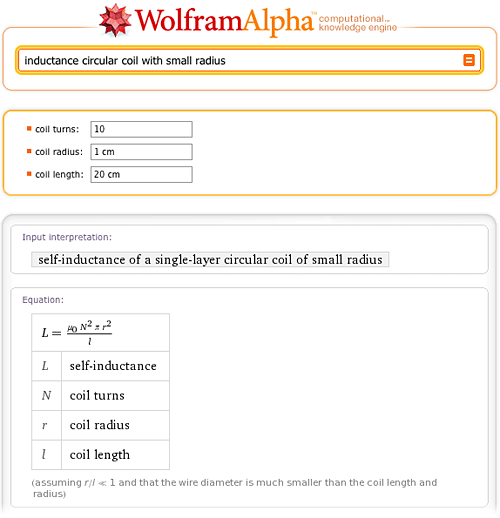While Wolfram|Alpha knows (and allows you to compute with) this simple formula, it also knows the correct general result. In fact, if you just ask Wolfram|Alpha for inductance circular coil, you will be simultaneously presented with two calculators: the one you know from your electromagnetics textbook (small-radius approximation) and the fully correct one. And not only can you compute the results both ways (and see that the results do differ slightly for the chosen parameters, but that the difference can get arbitrarily large), you can also click on the second “Show formula” link (near the bottom of the page on the right side) to see the exact result—which, as can be seen, contains two sorts of special functions, denoted E(m) and K(m) and known as elliptic integrals:

Because of special functions’ importance for the natural sciences, their numerical calculation and symbolic manipulation has always been a important part of Mathematica. In fact, Mathematica‘s coverage of special functions exceeds that of any other existing software package. In addition, The Wolfram Functions Site contains the largest published collection of formulas and identities for special functions—much larger than, say, the recently published Digital Library of Mathematical Functions. And because it is based on Mathematica and The Wolfram Functions Site, Wolfram|Alpha knows a lot about special functions such as Airy functions, Bessel functions, elliptic functions, hypergeometric functions—the list goes on and on. For all these functions, a variety of identities can be retrieved, including series expansions, integral representations, integral transforms, and many, many more.

Just entering the name of a special function in Wolfram|Alpha results in a variety of information and plots about that function, such as for the Airy function Ai(x):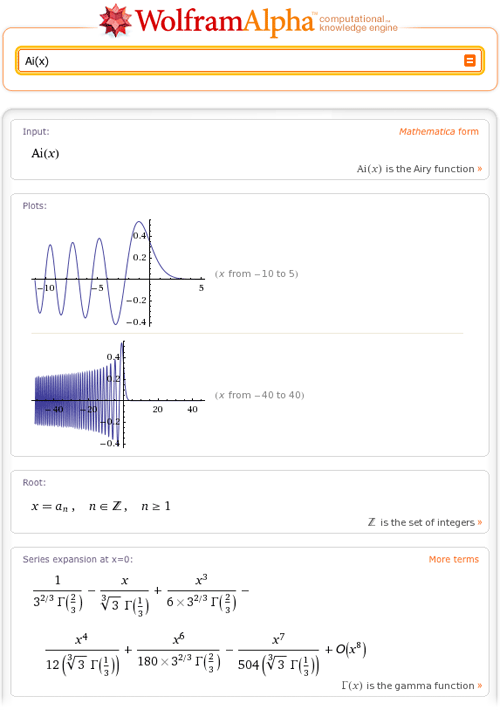One can also ask more detailed for information, such as integral representation of a LegendreQ function, series representation for the Riemann zeta function, or representations of ellipticK. Other examples: half angle formula for sn, dilog’s functional equations, integral representation hypergeometric2F1(3,4,5,(1+z)/2), and special cases of Jn(z).

Various more advanced identities are also known, to researchers and to Wolfram|Alpha. For example, the last few years have seen a dramatic increase in the interest in fractional derivatives, including in a recent prominent proof of the physical correctness of the famous Boltzmann equation. As you would hope and expect, Wolfram|Alpha can tell you various useful facts about such objects; here are the fractional derivatives of the logarithm: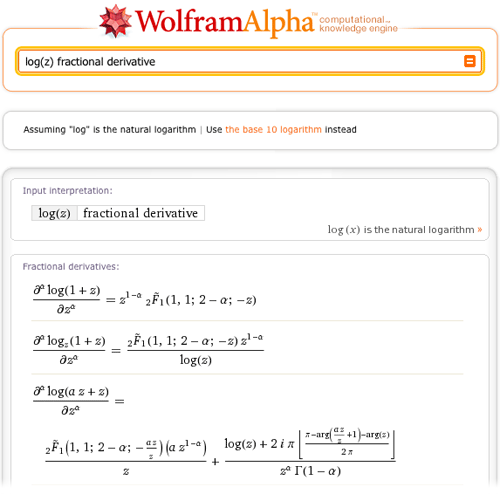Wolfram|Alpha also knows about so-called generalized functions (e.g. common limit representations of the Dirac delta function), about orthogonal polynomials (for instance the addition theorem for Jacobi polynomials or the recurrence relation for Hermite polynomials), and about many number-theoretic functions (such as how to write the nth prime number as a sum or various inequalities for the Euler phi function).

Of course, all the details that are available for special functions are also available for elementary functions and constants such asand e. For instance, try arcos(z) continued fraction representationsdifferential equation for logz expz sinz, nth-order symbolic derivatives of tan(x) or relations between sinx and tanx, and some useful sums that contain the binomial function.

Many identities for special functions and constants have names associated with the people who found them, such as various famousformulas, some classical Machin formulas, some modern BBP formulas, and a list of named identities for Fibonacci numbers:

While many more formulas for many more special functions are known to Wolfram|Alpha, the space in this blog is limited. But by all means feel free to explore the world of special functions further on your own with the help of Wolfram|Alpha.

Hi,

Could you add data sheets to Wolfram|Alpha for common Valve/Tube electronic parts, such as, for example:

* EL34
* EL84
* KT66
* KT88, i.e. http://en.wikipedia.org/wiki/KT88

To name but a few.

Cheers,
Edward.

Posted by Edward O'Callaghan June 15, 2010 at 10:27 am

Hello,

Great post! Really clear presentation of ideas and ubiquitous-but-unknown importance of special functions.

Cheers,
Mimi

Posted by Mimi Young June 15, 2010 at 10:46 am

Hi there,

log(z) fractional derivative works fine, but if one types

exp(z) fractional derivative

into Wolfram|Alpha, one sees the exp()-function only in a general series expression again. Why is this the case, is there no direct expression for the partial derivative of exp(z)?

Best regards
Udo.

Posted by U. Krause June 24, 2010 at 3:58 am

Very useful article!

Just one comment concerning the phrase

“Wolfram|Alpha knows a lot about special functions such as Airy functions, Bessel functions, elliptic functions, hypergeometric functions—the list goes on and on”

Clicking to “hypergeometric functions” link one gets basically WA examples on Gamma, Beta, error, Legendre etc. While it would be better to see power of WA for hypergeometric function itself. For instance give link to Hypergeometric2F1[a,b,c,z], or something similar.

Posted by sls July 2, 2010 at 11:49 am

Curious that WA only evaluates hypergeometric functions if entered with all commas, not the mixed comma-and-semicolon notation.

Posted by PM June 27, 2011 at 1:09 pm